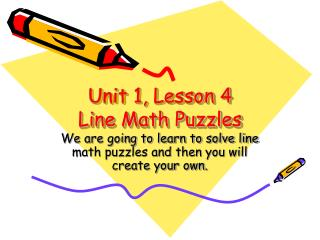DownloadDownload PresentationUnit 1, Lesson 4 Line Math Puzzles

# Unit 1, Lesson 4 Line Math Puzzles

Télécharger la présentation## Unit 1, Lesson 4 Line Math Puzzles

- - - - - - - - - - - - - - - - - - - - - - - - - - - E N D - - - - - - - - - - - - - - - - - - - - - - - - - - -
##### Presentation Transcript

1. Unit 1, Lesson 4 Line Math Puzzles We are going to learn to solve line math puzzles and then you will create your own.

2. Goals • Develop mathematical reasoning • Practice addition facts

3. Teaching the Activity • This line math puzzle is made of boxes connected with lines • What is the sum of each line? • What pattern do you see? • What number is in all three sums? • What other patterns do you see?

4. Line math puzzles • Each line of boxes connected by a dotted line in a line math puzzle has the same sum • Arrange the numbers on the Line Math Puzzles 1 and Line Math Puzzles 2 activity pages so that each line has the specified sum. • Write the number sentences on the side of the page next to the puzzles

5. Extension • Make your own design for the line and box structures of the puzzle • Create line math puzzles based on a new rule: Every line has a different sum • Use 10, 11, 12, 13, 14, 15, 16, 17, 18, 19, 20 to do a line math puzzle